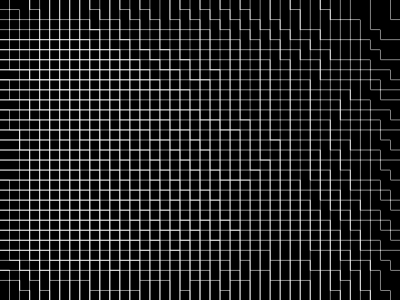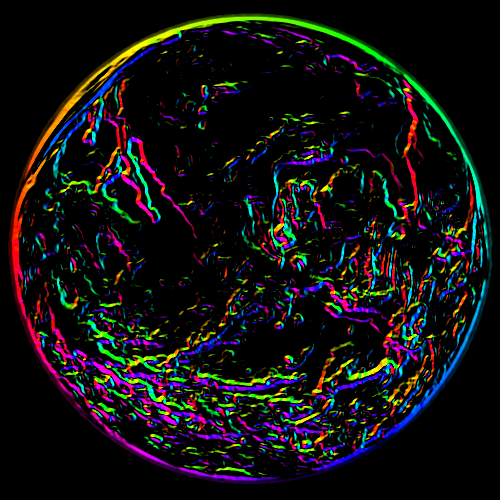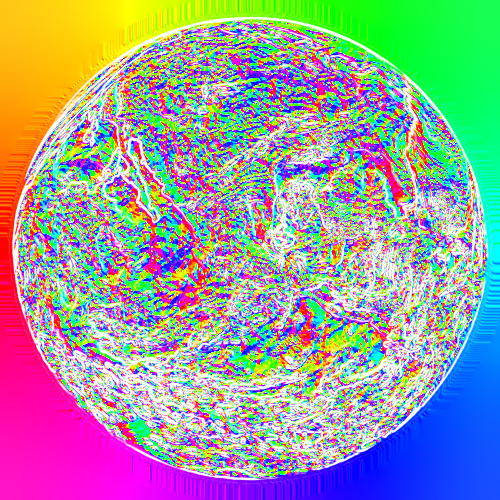G'MIC exercises

I was thinking of a different method entirely which involves splitting the channels but this might work. The Matlab code does seem a little tricky for me to understand though. I can sort out the if and for stuff just fine but I’m unfamiliar with matrices in G’MIC. map looks like it’s initially a two-dimensional matrix of zeros and I have no idea how it works. The stuff in the if structure is also difficult for me to understand. I also have no idea how vectorN works.

Mind you, I’ve found an extension of Cubehelix which allows for interpolation between any two different colours. It’s quickly becoming something that I don’t think that I can do because of how much technical stuff it requires.

Maybe `\$ gmic repeat 40 sp "\$>" done`
(but you have to remember there are 40 sample images right now, and this may be extended in the future)

This is something I have to fix ASAP, I guesshi, i-m new on this. i want to use ebsynth and dont know how to make the target gpos. do you know how can i use it to create this. Thank you so much. any advice?

@bazza me encataria hablar contigo, veo tus trabajos y es muy interesante lo que haces. he intentado entender un poco como crear la diferencia entre los gsec para crear el gpos. Cualquier ayuda o tip seria muy util para mi. muchisimas gracias de antemano.

graciasparece que lo genera usando algun tipo representación de un “flow” es como una transformación de una imagen a la otra. logre crear una versión usando deepmatch y deepflow no es igual, pero da buen resultado, pense que gmic podria hacer algo así.

Following up with my exploration of `gradient_norm` here and here

As I am having fun with G’MIC, I have been noticing that the appearance of `gradient_norm` heavily depends on the size (and contents) of the image.

This is a problem because what works for a small image won’t work for a large one. E.g., when I apply the same command to PlayRaw images, it doesn’t work because I have been playing with G’MIC `sample` images, which tend to be much smaller and more idealized.

One approach might be to resize the image. Take `sample flower` for example. The difference between #1 and #2 is that I doubled the size of #2 before applying `gradient_norm` and shrinking it back to size. (Actually, #1 vs #2 of `flower` might not be the best example; it still looks okay.)

With this strategy, I am able to thin the outline. So, I now know that I can manipulate the width of the outline by doing this. However, the details and intensities change resulting a different effect on the image, and this would compound as you chain more commands.

2 Likes

You’ve given me an idea for multi-scale gradient norms - I’ll see what I can do.

1 Like

To thin edges of gradient norm you can do this. It’s not perfect because the thin edges are not centred, but you may find it useful

``````-gradient_norm

--l[-1]
(1,-1)
-convolve[-2] [-1]
-rm[-1]
-c 0,255
-endl

--l[-2]
(1;-1)
-convolve[-2] [-1]
-rm[-1]
-c 0,255
-endl

-max[-1,-2]``````

Hmm, I am attempting to understand how G’MIC can create pattern, and it is my understanding that patterns can be defined by x-pixel, and y-pixel as well as width, and height. I am attempting to generate this pattern - https://forums.getpaint.net/topic/3691-vibrato-ymd080223/?_fromLogin=1 , but however, user-defined function is not getting me the answer I need. I am using user-defined function in order to understand how that works first.

However, I did found a cool idea, but it would require symmetrizing and the smooth modulo operation I just implemented for Krita.

Leaving this here for future reference : (x - y)/i * (x - i)/i * (﻿x + i)/i * (y - i)/i * (y + i)/i

And this here for future reference : http://users.cs.cf.ac.uk/Dave.Marshall/CM0268/PDF/10_CM0268_Audio_FX.pdf

EDIT: I realize I could use solidify filter approach instead to recreate vibrato. I think a lot of effect from the paint.net forum can be recreated with the help of solidify feature. I think I might create a thread just for that task.

Also, I really could use a way to assume the center of the image is 0 in G’MIC user-defined. Wow, this is hard, but learning…

K3DSurf to G’MIC

K3DSurf:
cos(x*y)

G’MIC:
cos((x-w/2)/w*(y-h/2)/h)

that means, for x is replaced by (x-w/2)/w, and y is replaced by (y-h/2)/h

And to get to -pi, pi coordinate for G’MIC, the end equation is:
cos(((x-w/2)/w) * pi * 2 * ((y-h/2)/h) * pi * 2)

Also, this equation might lead to Moire for paint.net to G’MIC
sin((((x-w/2)/w)pi2)^2+(((y-h/2)/w)pi2)^2)

sin((((x-w/2)/w) * pi * 2 * 10) ^ 2+(((y-h/2)/w) * pi * 2 * 10)^2) leads to a interesting moire. The 10 are adjustable.

Your rendering engine setting affects the moire result when zoomed away. Bilinear filtering leads me to apparent moire.

Are there two questions here? It’s a little hard for me to decipher what you’re asking at the beginning!

The question about input coordinates - if I had to convert some formula based on -1 to 1 domain, I would probably define another variable or vector first and work with that to simplify readability, e.g.

``````f "X=(x/w-.5)*2; Y=(y/h-.5)*2; cos(X*Y)"
``````

Just to restate what you probably already know: g’mic coords in a fill use 0 <= x < w, so quite often you need to convert to -1 to 1 or 0 to 1.

2 Likes

Yes, that is what I wanted. Now, I think I can find out what vibrato filter for paint . NET does.

I got this far for user-defined function :

``````f "X=(x/w-.5)*2; Y=(y/h-.5)*2; ;Z = (X - Y) * (X - 1) * (X + 1) * (Y - 1) * (Y + 1);1 - ( Z>.0001 ? Z : 1- Z*-1)"
``````

A few more tweak to the formula to add into modulo operation seen in paint .NET thread, and to add more modulo operation after the normalize would be enough to mimic Vibrato plugin for paint .NET.

``````- Normalize [Insert Function] * Z mod 256
``````

That’ll be what I’ll do.

Regarding the gradient norm stuff, here’s what I’ve got so far:

``````#@gui Gradient norm [JR's mod] : fx_jr_gradient_norm, fx_jr_gradient_norm_preview(1)
#@gui : Smoothness = float(0,0,10)
#@gui : Contrast = float(0.45,0,1.5)
#@gui : Min threshold = float(40,0,100)
#@gui : Max threshold = float(60,0,100)
#@gui : Negative = bool(0)
#@gui : Scale X = float(1,0,5)
#@gui : Scale Y = float(1,0,5)
#@gui : Interpolation = choice(2,"Nearest","Average","Bilinear","Bicubic")

if {\$8==3}
inter=5
else
inter={1+\$8}
fi
if {\$6!=1||\$7!=1}
ww={w}
hh={h}
shift. -0.5,-0.5,0,0,1
r. {max(1,\$6*w)},{max(1,\$7*h)},100%,100%,\$inter
fi
if {\$6!=1||\$7!=1}
r. \$ww,\$hh,100%,100%,\$inter
fi
``````It took me much longer than it should’ve to figure out that I had to shift it a little.

And of course, I thought I’d make it into something even bigger and include my fix for the gradient norm filter and a new fix for the direction2rgb filter. Turns out there was yet another missing `cut`.@Joan_Rake1

1. How did you arrive at the grid-like image?

2. The “embossed” lines radiating from the earth is neat. Would be even better if you could make the lines perpendicular to the tangent.

1. How did you arrive at the grid-like image?

This was just a simple and quite shallow gradient which I scaled up with nearest neighbour interpolation using GIMP - after which I applied the filter.

2. The “embossed” lines radiating from the earth is neat. Would be even better if you could make the lines perpendicular to the tangent.

It’s not intended to be an artistic filter, it’s more of a technical one. When the gradient norm is negative and the dir2rgb option is checked, it just computes the dir2rgb of what’s already there and anything which doesn’t seem to have a direction as such (which would normally appear black if one ran dir2rgb by itself) gets masked out as alpha and the alpha channel is removed using `fx_solidify_td`. Those ‘embossed lines’ are artefacts and nothing more, they can be removed by using the thresholds or the smoothness options.

I’ve got a newer version with some functional fixes which I’ll put up soon.

``````#@gui Vibrato Filter : fx_vibrato, fx_vibrato_preview(0)
#@gui : note = note("This filter is inspired by the Paint.NET plugin named Vibrato authored by MadJik for Paint.NET.")
#@gui : sep = separator()
#@gui : 1. X-Coordinate Pixel Based = float(1,0,2048)
#@gui : 2. Y-Coordinate Pixel Based = float(1,0,2048)
#@gui : 3. X-Coordinate Modulus Operation = float(1,0,32)
#@gui : 4. Y-Coordinate Modulus Operation = float(1,0,32)
#@gui : 5. Z-Modulus Operation = float(1,0,32)
#@gui : sep = separator(), Preview type = choice("Full","Forward horizontal","Forward vertical","Backward horizontal","Backward vertical","Duplicate top","Duplicate left","Duplicate bottom","Duplicate right","Duplicate horizontal","Duplicate vertical","Checkered","Checkered inverse")
#@gui : sep = separator(), note = note("<small>Author : <i>Reptorian</i>.      Latest update : <i>2018/09/22</i>.</small>")
fx_vibrato:
-normalize r.{{a = x+\$1<0 ? w - abs(x+\$1) : x + \$1},{b = y+\$2<0 ? w - abs(y+\$2) : y + \$2}, {c = a*\$3 mod (w+\$ 1)}, {d = b*\$4 mod (h+\$2)}, {X = (c/w-.5)*2}, {Y = (d/h-.5)*2},{ Z = (X - Y) * (X - 1) * (X + 1) * (Y - 1) * (Y + 1)},{1 - ( Z>.0001 ? Z : 1- Z*-1)}} n 0 255 mul \$5 mod 256
endl done
fx_vibrato_preview :
gui_split_preview "fx_vibrato \${1--2}",\$-1
``````

My first work into Vibrato filter. Not working at the moment. How to do the equivalent of what I did for user-defined filter?

Also, I think that a working base of it could be the main base for generating many different patterns found in paint . NET forum as all I need is to plug in X and Y for different filters.

I’ve tried to get your filter at least working, but not working as it should:

``````#@gui Vibrato Filter : fx_vibrato, fx_vibrato_preview(0)
#@gui : note = note("This filter is inspired by the Paint.NET plugin named Vibrato authored by MadJik for Paint.NET.")
#@gui : sep = separator()
#@gui : 1. X-Coordinate Pixel Based = float(1,0,2048)
#@gui : 2. Y-Coordinate Pixel Based = float(1,0,2048)
#@gui : 3. X-Coordinate Modulus Operation = float(1,0,32)
#@gui : 4. Y-Coordinate Modulus Operation = float(1,0,32)
#@gui : 5. Z-Modulus Operation = float(1,0,32)
#@gui : sep = separator(), Preview type = choice("Full","Forward horizontal","Forward vertical","Backward horizontal","Backward vertical","Duplicate top","Duplicate left","Duplicate bottom","Duplicate right","Duplicate horizontal","Duplicate vertical","Checkered","Checkered inverse")
#@gui : sep = separator(), note = note("<small>Author : <i>Reptorian</i>.      Latest update : <i>2018/09/22</i>.</small>")
fx_vibrato:
repeat \$! l[\$>]
if {x+\$1}
n 0,255 f "x+\$1<0?a=w-abs(x+\$1):a=x+\$1;y+\$2<0?b=w-abs(y+\$2):b=y+\$2;c=(a*\$3)%(w+\$1);d=(b*\$4)%(h+\$2);X=(c/w-.5)*2;Y=(d/h-.5)*2;Z=(X-Y)*(X-1)*(X+1)*(Y-1)*(Y+1);Z>.0001?1-Z:1-(-1*Z)" n 0,255 mul \$5 mod 256
fi endl done
fx_vibrato_preview :
gui_split_preview "fx_vibrato \${1--2}",\$-1
``````

From here on out all that we need to do is change the equation in the fill command.

Yeah, I noticed some few issues here and there. I’ll have to experiment if I want this filter to happen. I did left some transparency to see if I can get some of the channel mixing effect from the pdn filter. Also, preview doesn’t match the actual result.

EDIT:

Now I figured out the shift, and the scale factor.

f "l=0;
m=0;
n=1;
o=1;
a=(x+l<0?w-abs(x+l):x+l);
b=(y+m<0?h-abs(y+m):y+m);
c=(a * (1/n))%(w+l);
d=(b * (1/o))%(h+l);
X=(c/w-.5) * 2;
Y=(d/h-.5) * 2;
Z=(X-Y) * (X-1) * (X+1) * (Y-1) * (Y+1);
1-(Z>.0001?Z:1-Z * -1);
"

The modulo factor seem to be very difficult.

Aw man, there is no frac function in g’mic?! That would solve my issue.

You could use the wikipedia definition or use “abs(N)%1” depending on your needs. If there is a gmic equivalent I’m afraid I forgot it!

That’s not the same thing. frac (-.222) is 1-.222. Basically wanting a expression where it’s ignorant of negative number. Mod don’t work with negative numbers.